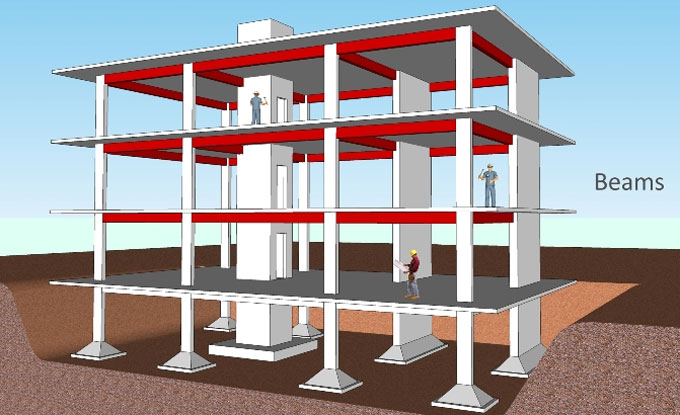# Design of Beam1. Estimation of constants. -For the specified set of stresses, determine KC, JC and RC.

2. Kc= M~cbc/M~cbc+~st

3. Jc= 1-(Kc/3)

4. Rc= 0.5*L*Kc Jc

5. Estimation of bending moment. Suppose appropriate values of overall depth and breadth of beam, and find out the effective span. Work out the self-weight and total U.D.L. and maximum bending moment in the beam.

6. Design of the section. Workout the effective depth of the beam with the following expression:

7. d= [(M)/ (RC*b)] 0.5

8. Reinforcement. Workout the area with the formula.

9. Shear Reinforcement. Workout the maximum shear force in the beam.

10. Verify for Development length at the end.

11. Ld<= (M1/V) +L0.

Design of column:

1. Find out the allowable stresses in concrete, longitudinal bars and ties.
2. Determine the super impose load that should be borne by the column.
3. Find out the area from the following expression - P= ~cc *Ac+~sc*Asc.
4. After getting details about the area, find out the dimensions of column. If it is a square of side b, then b=Ag.
5. For the specified end conditions, find out the effective length of column. Measure lef/b ratio to determine whether the column is short or long.
6. If lef/b ratio<12 it will be designed as short column or else as long column; define the area of steel Asc.
7. Determine the diameters of bars utilized as ties and find out its pitch according to the rules.

DESIGN OF FOOTING: The width B of the footing will obviously be equivalent to [W+W"]/qo. The thickness is measured based on the bending moment as well as punching shear.

1. Depth for bending moment.
d= [M/B*Rc] 0.5
2. Depth for shear.
3. tv=V/B*d
4. Steel Reinforcement. Ast=M/tjcd